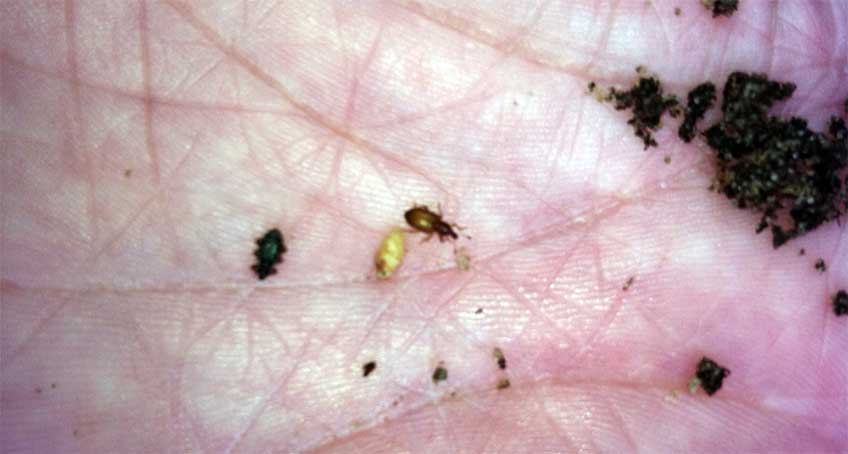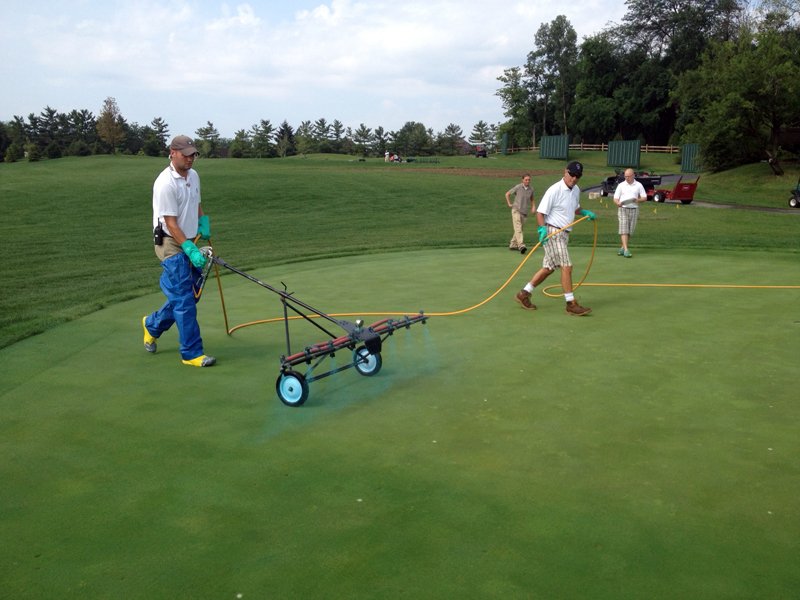Field Insights Blog | GreenCast | Syngenta
Field Insights Blog | GreenCast | Syngenta# Growing Degree Days

As spring rapidly approaches, and with the threat of annual bluegrass weevil (ABW) on the horizon, I thought I would comment on growing degree days (GDD) since they are used in WeevilTrak. You can also sign up for GDD alerts at GreenCastOnline.comPhotograph 1.  The ABW (adults shown above) life cycle can be predicted based on growing-degree days. Most turf and ornamental insects life cycles can bredicted by growing degree day models.

Temperature is the driving force for all biological activity. As such, for many organisms, their growth, development and reproduction is predictable based on temperature. A degree day, also known as growing degree days (GDDs) and heat units, is a measure of heat above a threshold for one day. Growing degree accumulation is a reflection of the accumulated degree days, or GDDs or heat units above a threshold for consecutive 24-hour days. An important aspect of GDD is that no units (ex. grams, liters/hectare, etc.) are associated with the value. The accumulated GDD values are correlated with an event in an organism’s life.Photograph 2. Growing degree day models have been developed recently that aid in the application timing of plant growth regulators.

Calculating GDDs

There are three ways to calculate GDDs The most common is the Average Method where the daily minimum temperature is added to the maximum temperature for that day and then divided by two. The base temperature, which can vary depending on the plant model, is subtracted from the average. If the calculated GDD is greater than 0 it is added to the cumulative total that has occurred since the start date. If the GDD is less than zero it is set to zero. The base temperature can vary widely depending on the GDD model ranging from 0 to 13° C (32 to 55° F) with some models. The most common base temperature used is 10° C (50° F)

The second method is called the Modified Average Method which calculates GDD the same way as the Average Method except if the minimum temperature is below the base temperature; the base temperature is used as the minimum temperature in the calculation. This calculation has an advantage over the Average Method occurs when temperatures fluctuate above and below the base temperature, which often occurs during the early spring. When fluctuation occurs above and below the base temperature the Average Method underestimates the number of GDDs because even some biological activity occurs above the base temperature. From an application perspective, for spring timing of a mefluidide (plant growth regulator) application for Poa annua seedhead control, an appropriate method for GDD calculation would be the Modified Average Method.

The Modified Sine Curve Method is the third and most accurate means of calculating GDD. It is based on the assumption that the daily diurnal temperature pattern is similar to a sine curve. The GDD calculated is the area under the curve. The calculation done with the Modified Sine Curve compared to the previous two methods is considerably more complex and often requires a computer program.

The Modified Sine Curve Method has an additional benefit of accounting for a high temperature threshold. In other words an upper temperature limit can be set where GDD are calculated to the threshold and not above.

The last comment I would like to make on GDDs is in reference to the start date. With all GDD models, a set start date is given which is sometimes referred to as biofix. The common start date is January 1, but again this can vary depending on the model. Early GDD models that predicted annual bluegrass or Kentucky bluegrass seedhead emergence had a start date of April 1 and March 1, respectively (northern hemisphere). However, many of the insect models and weed models begin with January 1.Dr. Karl Danneberger is a professor of Turfgrass Science at The Ohio State University. Dr. Danneberger's contact information can be found here. You may also follow Dr. Danneberger on Twitter: